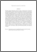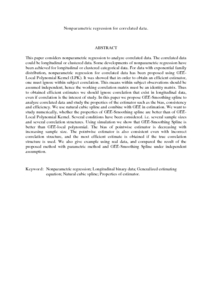# Nonparametric regression for correlated data.

## Citation

Ibrahim, Noor Akma and Suliadi, Suliadi (2009) Nonparametric regression for correlated data. WSEAS Transactions on Mathematics, 8 (7). pp. 331-340. ISSN 1109-2769

## Abstract

This paper considers nonparametric regression to analyze correlated data. The correlated data could be longitudinal or clustered data. Some developments of nonparametric regression have been achieved for longitudinal or clustered categorical data. For data with exponential family distribution, nonparametric regression for correlated data has been proposed using GEE-Local Polynomial Kernel (LPK). It was showed that in order to obtain an efficient estimator, one must ignore within subject correlation. This means within subject observations should be assumed independent, hence the working correlation matrix must be an identity matrix. Thus to obtained efficient estimates we should ignore correlation that exist in longitudinal data, even if correlation is the interest of study. In this paper we propose GEE-Smoothing spline to analyze correlated data and study the properties of the estimator such as the bias, consistency and efficiency. We use natural cubic spline and combine with GEE in estimation. We want to study numerically, whether the properties of GEE-Smoothing spline are better than of GEE-Local Polynomial Kernel. Several conditions have been considered. i.e. several sample sizes and several correlation structures. Using simulation we show that GEE-Smoothing Spline is better than GEE-local polynomial. The bias of pointwise estimator is decreasing with increasing sample size. The pointwise estimator is also consistent even with incorrect correlation structure, and the most efficient estimate is obtained if the true correlation structure is used. We also give example using real data, and compared the result of the proposed method with parametric method and GEE-Smoothing Spline under independent assumption.Preview
PDF ( Abstract)
Nonparametric regression for correlated data.pdfView Item